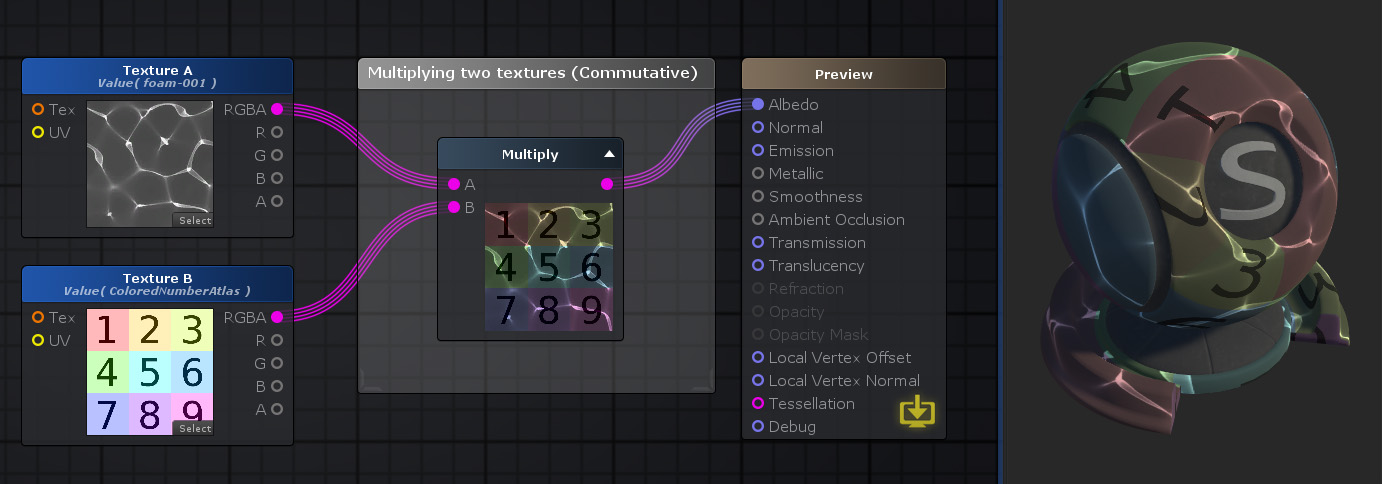Multiply Node

The Multiply Node ( shortcut: M key ) does an arithmetic multiplication of two or more values, up to 10, or ( A * B * ... ). By default the node only shows two input ports so if the addition of more than two values is needed a new port is dynamically added whenever an output port wire is picked up. These extra inputs are also dynamically removed if they aren't connected. This node also handles matrix multiplication. When that's the case the node no longer allows extra ports to prevent wrong matrices operations.

Multiplication between multi-channel data types are done per-channel. If A and B have different channel amounts a cast is done to match the one with the most amount of channels.

Note: Multiplication is commutative so value's order is not important but, matrix multiplication is not commutative and order is important. Keep that in mind when doing matrix multiplications.Nodes used: Texture Sample, Multiply

Node Parameter Description Default Value
A The first value. Only visible if the respective input port is not connected. 0
B The second value. Only visible if the respective input port is not connected. 0

Input Port Description Type
A The first value Float 
B The second value Float 
# The #n-th value, up to 10. Only visible when already connected or when you pick up an output wire connection. Float 

1. ^ Port automatically adapts to all connection types except Sampler types.